# 第一题 改错题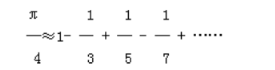modi.c文件代码如下：

#include <math.h>
#include <stdio.h>
/************下一行有错************/
fun(float num)
{
int s,n;
float t,pi;

t=1;pi=0;n=1;s=1;
/************下一行有错************/
while(t>=num)
{
pi=pi+t;
n=n+2;
s=-s;
/************下一行有错************/
t=s/n;
}
pi=pi*4;
return pi;
}

main()
{
float n1,n2;
printf("Enter a float number:");
/************下一行有错************/
scanf("%d",&n1);
n2=fun(n1);
printf("%6.4f\n",n2);
}



#include <math.h>
#include <stdio.h>
/************下一行有错************/
float fun(float num)
{
int s,n;
float t,pi;

t=1;pi=0;n=1;s=1;
/************下一行有错************/
while(t>=num||(-t)>=num)
{
pi=pi+t;
n=n+2;
s=-s;
/************下一行有错************/
t=(float)s/(float)n;
}
pi=pi*4;
return pi;
}

main()
{
float n1,n2;
printf("Enter a float number:");
/************下一行有错************/
scanf("%f",&n1);
n2=fun(n1);
printf("%6.4f\n",n2);
}


• return了一个浮点数，所以函数头前需要加上float表明返回值的类型
• t的循环判断条件有误，其实也可以使用fabs()函数来直接处理
• sn都是int型变量，除出来的数据必然是int型，只不过赋值给t时强制转float型，所以t值小数点后均为0，需要在计算之前强制转一下sn的类型
• float型不能使用%d，改用%f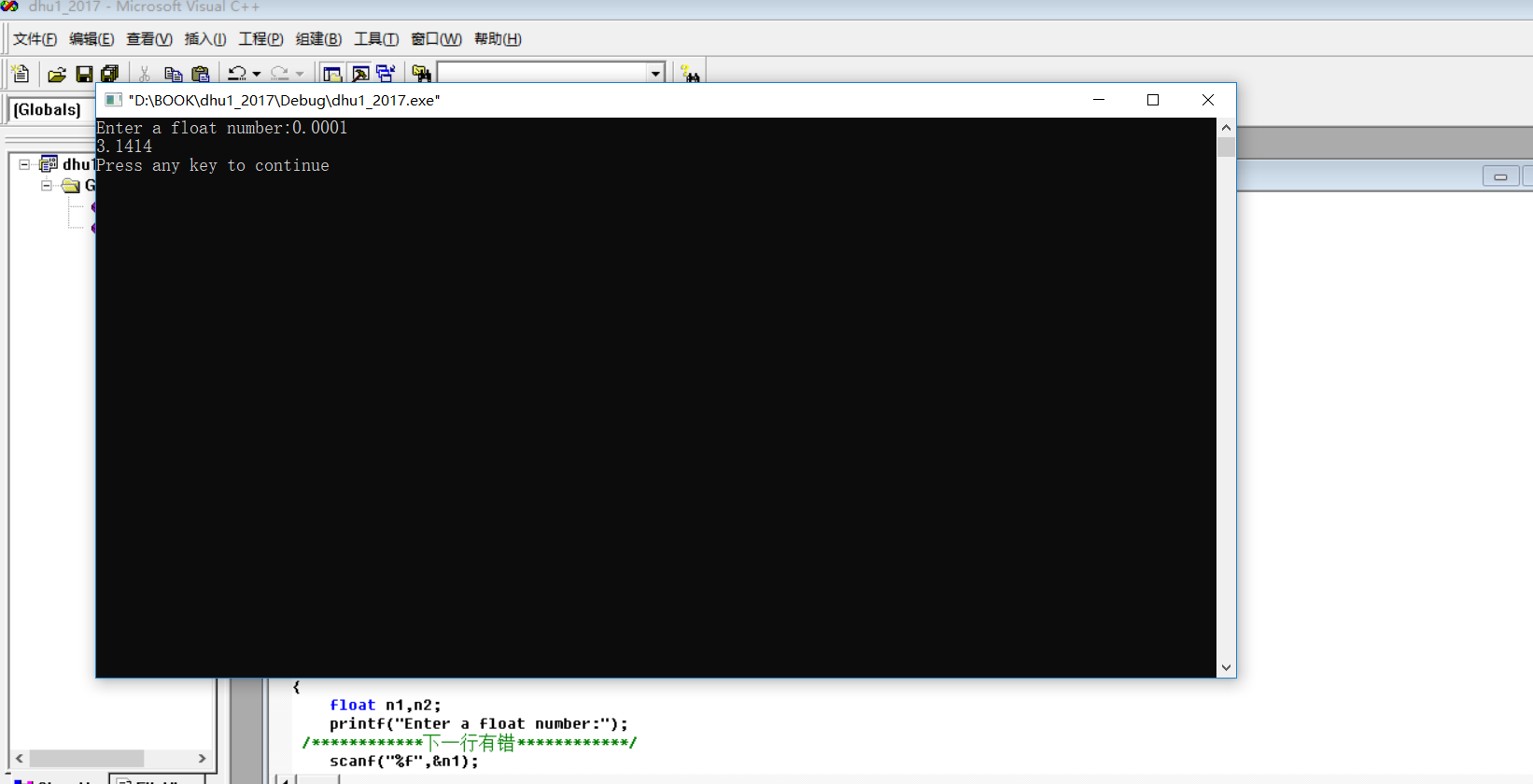# 第二题 补全代码题

159、168、177、186、195、249、258、267、276、285、294、339、348、357、366、375、384、393、429、438、447、456、 465、474、483、492。共有26个。

#include <stdio.h>
fun(int x)
{
int n, s1, s2, s3, t;
n = __1__;
t = 100;
while (t <= __2__)
{
s1 = t % 10;
s2 = (t / 10) % 10;
s3 = t / 100;
if (s1 + s2 + s3 == 15)
{
printf("%d ", t);
n++;
}
__3__;
}
return n;
}
main()
{
int x = -1;
while (x > 999 || x < 100)
{
scanf("%d", &x);
}
printf("\nThe result is: %d\n", fun(x));
}



#include <stdio.h>
fun(int x)
{
int n, s1, s2, s3, t;
n = 0;
t = 100;
while (t <= x)
{
s1 = t % 10;
s2 = (t / 10) % 10;
s3 = t / 100;
if (s1 + s2 + s3 == 15)
{
printf("%d ", t);
n++;
}
t = t+1;
}
return n;
}
main()
{
int x = -1;
while (x > 999 || x < 100)
{
scanf("%d", &x);
}
printf("\nThe result is: %d\n", fun(x));
}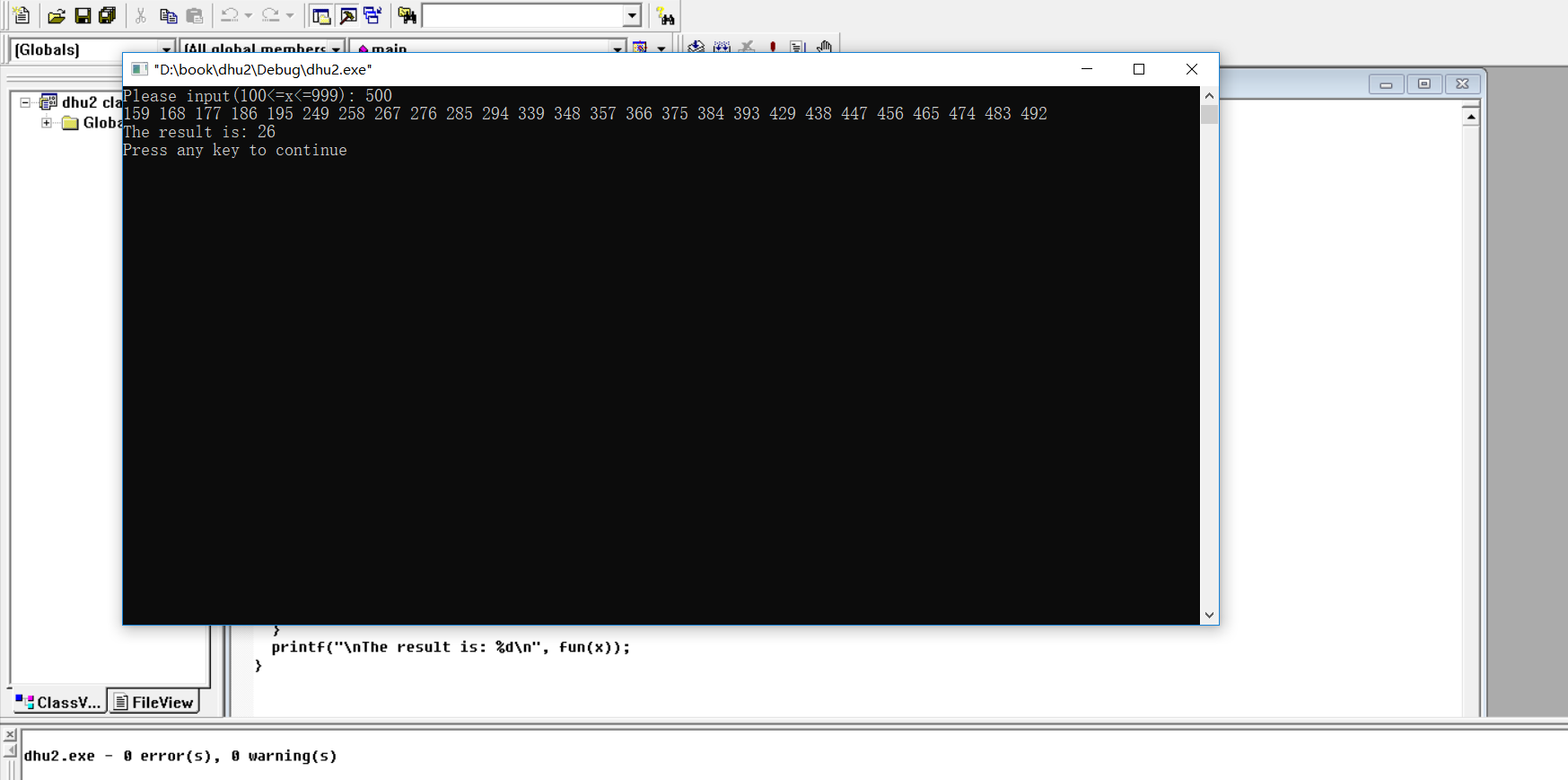这个就很简单了，没什么好解释的了

# 第三题 补全代码题

32486和12345

44831

blank2.c代码如下：

#include  <stdio.h>
#include  <string.h>
#include  <ctype.h>

int  strToNumber( char  s[] )
{
int  d=0;
int i=0;
while(s[i]!='\0')
if(isdigit( s[i]))
{
d=d*10+s[i]-__1__;
__2__;
}
return  d;
}
int  add( char  a[], char  b[] )
{
return  __3__;
}
main()
{
char  s1,s2;
printf("Input  string  s1 : ");
gets(s1);
printf("Input  string  s2 : ");
gets(s2);
printf("The result is:  %ld\n", add(s1,s2) );
}


#include  <stdio.h>
#include  <string.h>
#include  <ctype.h>

int  strToNumber( char  s[] )
{
int  d=0;
int i=0;
while(s[i]!='\0')
if(isdigit( s[i]))
{
d=d*10+s[i]-48;
i++;
}
return  d;
}
int  add( char  a[], char  b[] )
{
return  strToNumber(a)+strToNumber(b);
}
main()
{
char  s1,s2;

printf("Input  string  s1 : ");
gets(s1);
printf("Input  string  s2 : ");
gets(s2);
printf("The result is:  %ld\n", add(s1,s2) );
}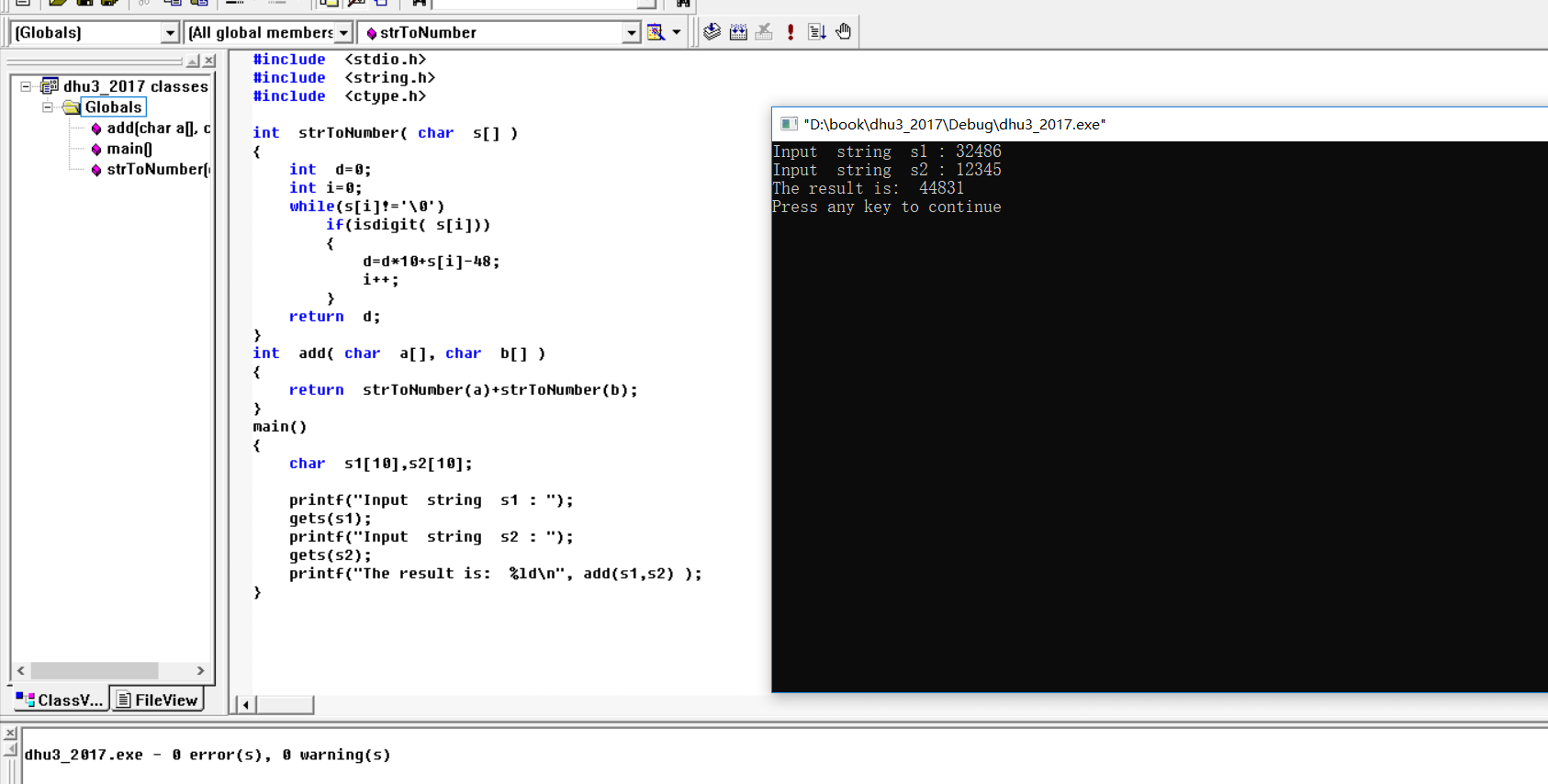# 第四题 编程题

fun函数的功能是：传入两个形参，一个是数组data，一个是n的值，经过计算，返回剔除 重复数字后剩下的数字的个数。

prog1.c如下：

#include <stdio.h>
NONO();
/*

*/
int fun(int array[],int n)
{

}
int main()
{
int data;//数组长度不超过200000
int n,count,i;
scanf("%d", &n);
for(i=0; i<n; i++)  //data数组实际存放n个元素
scanf("%d", &data[i]);
count=fun(data,n);
printf("%d\n", count);
NONO();
return 0;
}
NONO()
{//本函数用于辅助教师判卷，不需要阅读其中代码，也请不要改动其中代码。
FILE *rf,*wf ;
int a, i, j, p, n ;
rf = fopen("bc2.in", "r") ;
wf = fopen("p1.out", "w") ;
for(i = 0 ; i < 6 ; i++)
{
fscanf(rf, "%d", &n) ;
for(j = 0 ; j < n ; j++) fscanf(rf, "%d", &a[j]) ;
p=fun(a, n) ;
fprintf(wf, "%d\n", p) ;
}
fclose(rf) ;
fclose(wf) ;
}


#include <stdio.h>
NONO();
/*

*/
int fun(int array[],int n)
{
int flag = {0};
for(int i =0;i<n;i++){
flag[array[i]]++;
}
int sum = 0;
for(int i = 0;i<=32767;i++){
if(flag[i]!=0){
sum++;
}
}
return sum;
/*也可以稍微优化一下，如下：
int flag = {0};
int sum = 0;
for(int i =0;i<n;i++){
if(flag[array[i]]==0){
sum++;
}
flag[array[i]]++;
}
return sum;
*/
}
int main()
{
int data;//数组长度不超过200000
int n,count,i;
scanf("%d", &n);
for(i=0; i<n; i++)  //data数组实际存放n个元素
scanf("%d", &data[i]);
count=fun(data,n);
printf("%d\n", count);
NONO();
return 0;
}
NONO()
{//本函数用于辅助教师判卷，不需要阅读其中代码，也请不要改动其中代码。
FILE *rf,*wf ;
int a, i, j, p, n ;
rf = fopen("bc2.in", "r") ;
wf = fopen("p1.out", "w") ;
for(i = 0 ; i < 6 ; i++)
{
fscanf(rf, "%d", &n) ;
for(j = 0 ; j < n ; j++) fscanf(rf, "%d", &a[j]) ;
p=fun(a, n) ;
fprintf(wf, "%d\n", p) ;
}
fclose(rf) ;
fclose(wf) ;
}


# 第五题 编程题

5 3 4 2 4 1

main函数中，首先输入n，表示共有n个导弹，然后读入导弹的高度到数组a中。 fun函数接受a数组和n两个参数，计算需要的拦截系统的数目，并返回该结果。请完成fun 函数。

6
5 3 4 2 3 1



#include<stdio.h>
#include<string.h>
#include<stdlib.h>
NONO();
int  fun(int  height[], int  n)
{

}
int main ()
{
int n , a;
int i, k, total;
scanf ( "%d", &n );
for ( i=0; i < n; i++ )
{
scanf ( "%d", &a[i] );
}
total = fun(a, n);
printf("The number is: %d", total);
NONO();
return 0;
}
NONO()
{//本函数用于辅助教师判卷，不需要阅读其中代码，也请不要改动其中代码。
FILE *rf,*wf ;
int a, i, j, p, n ;
rf = fopen("bc.in", "r") ;
wf = fopen("p2.out", "w") ;
for(i = 0 ; i < 6 ; i++)
{
fscanf(rf, "%d", &n) ;
for(j = 0 ; j < n ; j++) fscanf(rf, "%d", &a[j]) ;
p=fun(a, n) ;
fprintf(wf, "%d\n", p) ;
}
fclose(rf) ;
fclose(wf) ;
}


#include<stdio.h>
#include<string.h>
#include<stdlib.h>
NONO();
int  fun(int  height[], int  n)
{
int point={0};
int k = 0;
for(int i =0;i <n;i++){
for(int j=0;j<n;j++){
if(point[j]>height[i]){
point[j] = height[i];
break;
}
else if(point[j]==0){
point[j] = height[i];
k++;
break;
}
}
}

return k;
}
int main ()
{
int n , a;
int i, k, total;
scanf ( "%d", &n );
for ( i=0; i < n; i++ )
{
scanf ( "%d", &a[i] );
}
total = fun(a, n);
printf("The number is: %d", total);
NONO();
return 0;
}

NONO()
{//本函数用于辅助教师判卷，不需要阅读其中代码，也请不要改动其中代码。
FILE *rf,*wf ;
int a, i, j, p, n ;
rf = fopen("bc.in", "r") ;
wf = fopen("p2.out", "w") ;
for(i = 0 ; i < 6 ; i++)
{
fscanf(rf, "%d", &n) ;
for(j = 0 ; j < n ; j++) fscanf(rf, "%d", &a[j]) ;
p=fun(a, n) ;
fprintf(wf, "%d\n", p) ;
}
fclose(rf) ;
fclose(wf) ;
}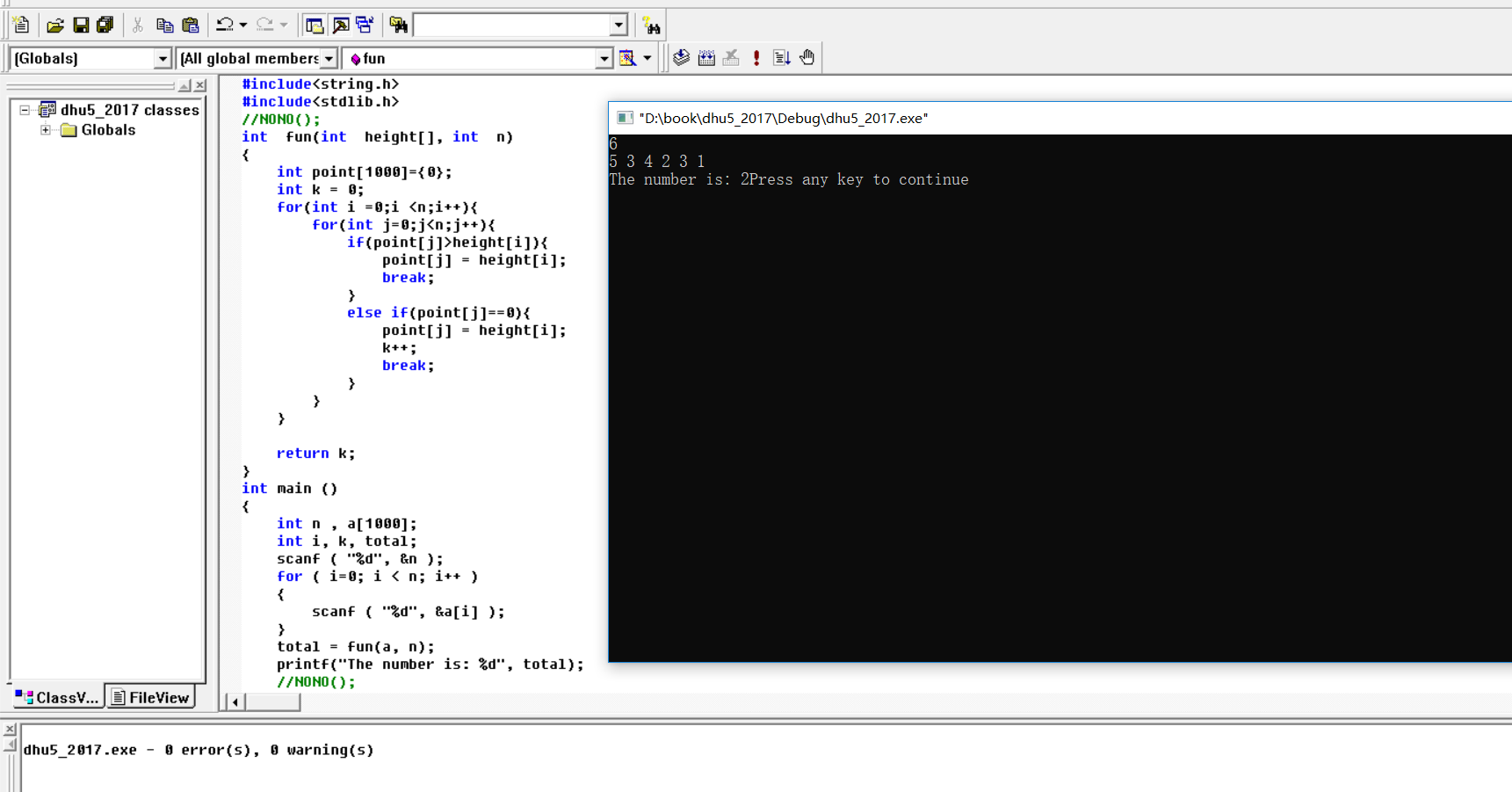# 第六题 编程题

struct PTNode {
char data; //结点数据域
int parent; //结点双亲在数组中的位置
};


#define MAX_TREE_SIZE 100
struct PTree {
struct PTNode nodes[MAX_TREE_SIZE]; //存储树中所有结点
int n; //树中共有 n 个结点，n 不超过 100
}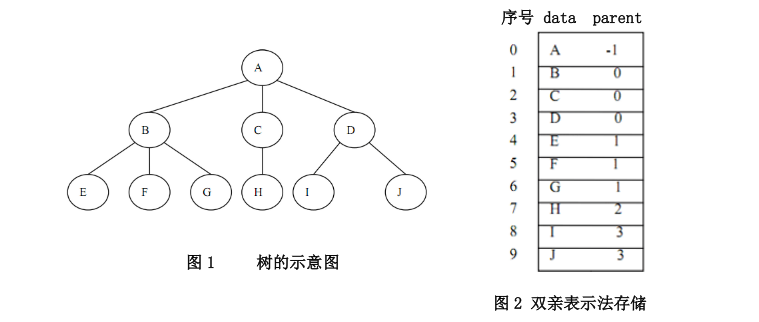已知一棵树已存储为以上形式，请编写函数 GetNearestCommonGrand，查找给定的两个
（不相同的）结点最近的共同祖先。GetNearestCommonGrand 的函数原型为： char GetNearestCommonGrand(struct PTree T, char nodeData1, char nodeData2)

T：保存了树中结点数目及图 2 所示的结点数组
nodeData1,nodeData2：给定的两个结点的数据（输入时保证这两个结点存在）。

prog3.c 中，struct PTree CreateTree()函数用于从键盘输入树的双亲表示法的信息，创 建一棵树。输入的第一个数 n 表示树中结点数，此后有 n 行输入，每行表示一个结点的信 息，其中第一个信息为结点的数据，第二个信息为结点的双亲结点在数组中的位置。 在 main 函数中还需要输入两个结点的字符数据，查询这两个结点的最近共同祖先.

10
a -1
b 0
c 0
d 0
e 1
f 1
g 1
h 2
i 3
j 3
g h

8
a -1
b 0
e 1
h 6
c 0
d 0
f 5
g 5
g h

prog3.c 源码如下：

#include <stdio.h>
#define MAX_TREE_SIZE 100
void NONO();
struct PTNode /*树的一个结点*/
{
char data;
int parent; /* 双亲位置域 */
};
struct PTree
{
struct PTNode nodes[MAX_TREE_SIZE];
int n; /* 结点数 */
};
/*

*/
char GetNearestCommonGrand(struct PTree T, char nodeData1, char nodeData2)
{

}
struct PTree CreateTree()
{
int i,n;
int parentId;
char ch;
struct PTree newTree;
scanf("%d", &n);
newTree.n=n;
for (i = 0; i < n; i++)
{
scanf(" %c%d", &ch, &parentId);
newTree.nodes[i].data=ch;
newTree.nodes[i].parent=parentId;
}
return newTree;
}
int main()
{
struct PTree aTree;
char node1, node2, nodeGrand;
aTree = CreateTree();
scanf(" %c %c", &node1, &node2);
nodeGrand= GetNearestCommonGrand (aTree, node1, node2);
printf("%c\n", nodeGrand);
NONO();
return 0;
}
void NONO()
{//本函数用于辅助教师判卷，不需要阅读其中代码，也请不要改动其中代码。
FILE *fp, *wf ;
int i,j,n;
char ch;
int parentId;
char node1, node2, nodeGrand;
struct PTree newTree;
fp = fopen("p3.in","r");
wf = fopen("p3.out","w");
for(i = 0 ; i < 6 ; i++)
{
fscanf(fp, "%d", &n);
newTree.n=n;
for (j = 0; j < n; j++)
{
fscanf(fp," %c%d", &ch, &parentId);
newTree.nodes[j].data=ch;
newTree.nodes[j].parent=parentId;
}
fscanf(fp," %c %c", &node1, &node2);
nodeGrand= GetNearestCommonGrand (newTree, node1, node2);
fprintf(wf, "%c\n", nodeGrand);
}
fclose(fp);
fclose(wf);
}


#include <stdio.h>
#define MAX_TREE_SIZE 100
void NONO();
struct PTNode /*树的一个结点*/
{
char data;
int parent; /* 双亲位置域 */
};
struct PTree
{
struct PTNode nodes[MAX_TREE_SIZE];
int n; /* 结点数 */
};
/*
将两个节点的双亲节点直到根节点全部存入数组，再从根节点开始对比，
直至找到不一样的节点，此处回退一个即为最近共同祖先

*/
char GetNearestCommonGrand(struct PTree T, char nodeData1, char nodeData2)
{
int tree1,tree2;
int i,j,m,n,flag1,flag2;
for(i =0;i<T.n;i++){
if(T.nodes[i].data == nodeData1){
flag1 = i;
}
else if(T.nodes[i].data == nodeData2){
flag2 = i;
}
}
i = 0;
tree2[i] = flag1;
i++;
j = flag1;
while(T.nodes[j].parent!=-1){
tree1[i]= T.nodes[j].parent;
i++;
j = T.nodes[j].parent;;
}
m = i-1;
i = 0;
tree2[i] = flag2;
i++;
j = flag2;
while(T.nodes[j].parent!=-1){
tree2[i]= T.nodes[j].parent;
i++;
j = T.nodes[j].parent;
}
n = i-1;
while(tree1[m]==tree2[n]){
m--;
n--;
}
return T.nodes[tree2[n+1]].data;

}
struct PTree CreateTree()
{
int i,n;
int parentId;
char ch;
struct PTree newTree;
scanf("%d", &n);
newTree.n=n;
for (i = 0; i < n; i++)
{
scanf(" %c%d", &ch, &parentId);
newTree.nodes[i].data=ch;
newTree.nodes[i].parent=parentId;
}
return newTree;
}
int main()
{
struct PTree aTree;
char node1, node2, nodeGrand;
aTree = CreateTree();
scanf(" %c %c", &node1, &node2);
nodeGrand= GetNearestCommonGrand (aTree, node1, node2);
printf("%c\n", nodeGrand);
NONO();
return 0;
}
void NONO()
{//本函数用于辅助教师判卷，不需要阅读其中代码，也请不要改动其中代码。
FILE *fp, *wf ;
int i,j,n;
char ch;
int parentId;
char node1, node2, nodeGrand;
struct PTree newTree;
fp = fopen("p3.in","r");
wf = fopen("p3.out","w");
for(i = 0 ; i < 6 ; i++)
{
fscanf(fp, "%d", &n);
newTree.n=n;
for (j = 0; j < n; j++)
{
fscanf(fp," %c%d", &ch, &parentId);
newTree.nodes[j].data=ch;
newTree.nodes[j].parent=parentId;
}
fscanf(fp," %c %c", &node1, &node2);
nodeGrand= GetNearestCommonGrand (newTree, node1, node2);
fprintf(wf, "%c\n", nodeGrand);
}
fclose(fp);
fclose(wf);
}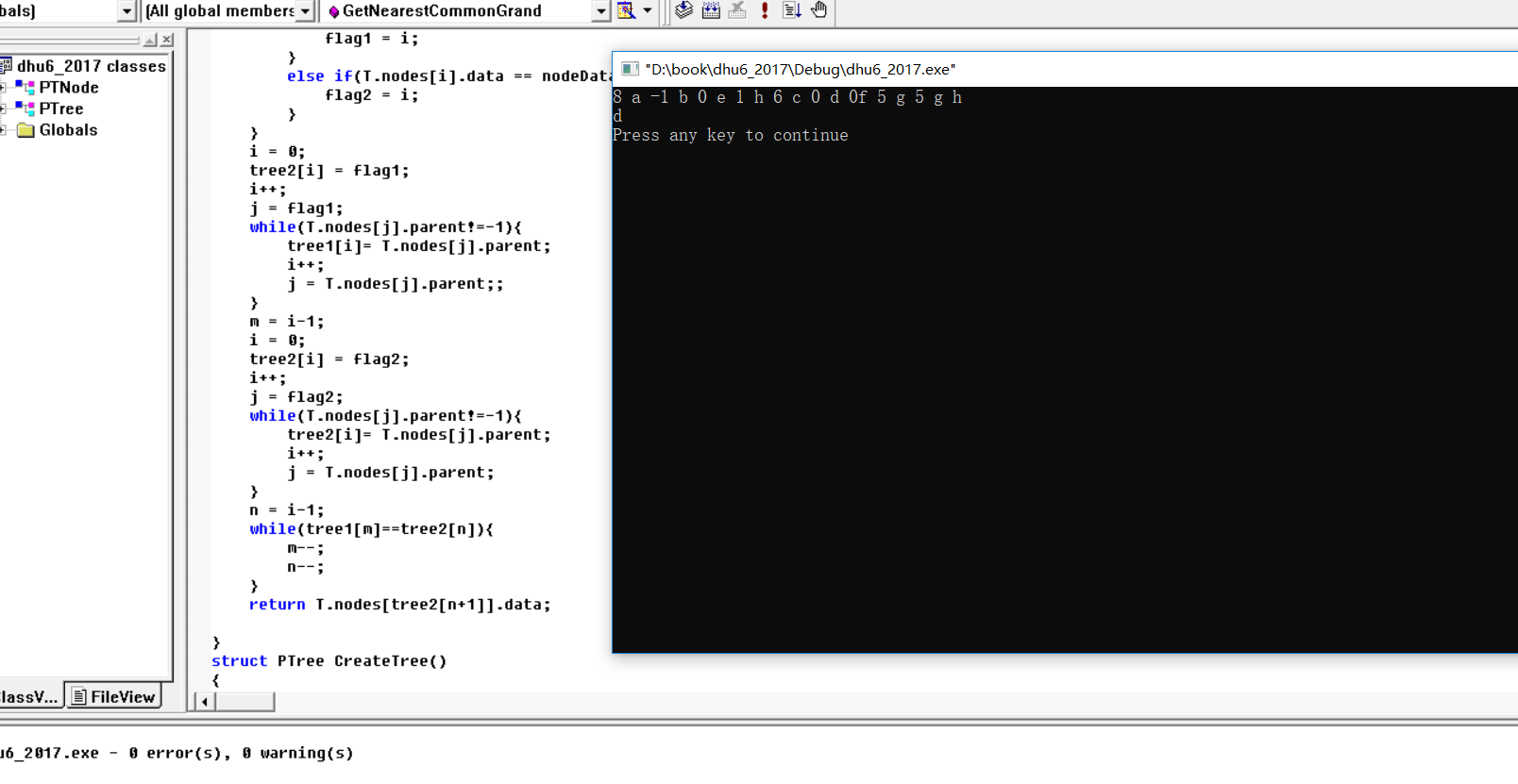# 总结

• 总体难度来说，中下难度
• 调试的时候NONO函数这种先注释掉，调试完再恢复
• vc++不怎么会用，摸索了半天，这里需要注意的是一个报错，fatal error C1010: unexpected end of file while looking for precompiled head

1.右键单击项目工程中的cpp文件，在菜单Project->Settings->C/C++->Precompile Header，设置为第一项：Not using precompile headers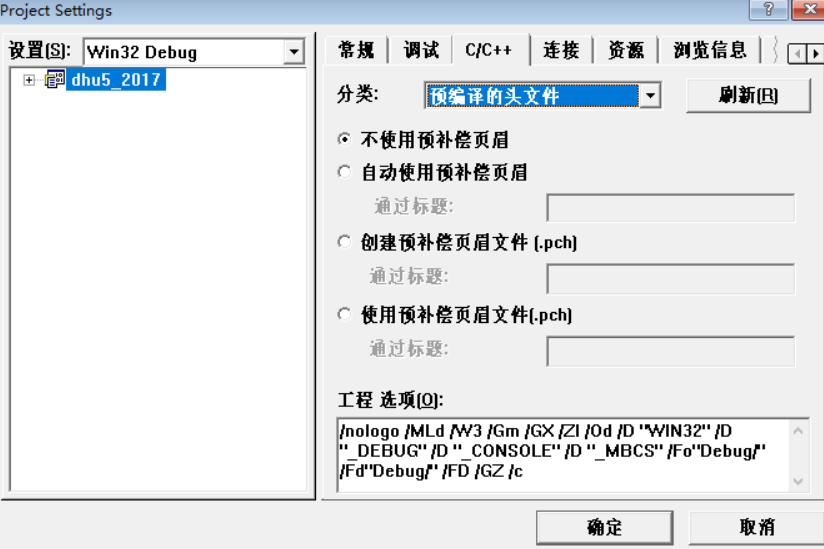2.在.c文件开头添加包含文件stdafx.h

#include"stdafx.h"


03-181156

#### 复试上机刷题3 东华18上机真题06-18271

#### C语言编程题——下列给定的程序中，函数fun的功能是：为一个偶数寻找两个素数，这两个素数之和等于该偶数，并将这两个素数通过形参指针传回主函数。

03-21512

#### 杭电2018复试上机真题

02-241万+

#### 输入一个字符串,按字典序打印出该字符串中字符的所有排列。例如输入字符串abc,则打印出由字符a,b,c所能排列出来的所有字符串（剑指offer）

08-05855

#### 牛客网—计算机历年考研复试上机刷题记录

12-211428

#### 数据结构上机测试试题【数据结构】

03-30511

#### 复试上机题集（2）

02-17136

#### 东华2017复试上机真题

03-30606

#### 复试上机题集（5）

02-093113

#### 计算机二级C语言程序修改题+答案解析+源代码（一）

04-102168

#### 将S 所指字符串中的数字移到字符串后面，相对次序不变。例：s=“a1b2c3d4efg” fun（s）="abcdefg1234"

02-131222

#### 计算机二级C语言程序填空题+程序修改+程序设计题答案解析（一）

03-09113

#### 复旦大学2014复试上机第二题——字符串的编辑距离（动态规划）

01-15168

#### C/C++学习指南全套教程

09-11©️2020 CSDN 皮肤主题: 技术工厂 设计师: CSDN官方博客点击重新获取扫码支付1.余额是钱包充值的虚拟货币，按照1:1的比例进行支付金额的抵扣。
2.余额无法直接购买下载，可以购买VIP、C币套餐、付费专栏及课程。余额充值